# Decimals

Here we will learn about decimals including how to add decimals, subtract decimals, multiply decimals and divide decimals.

There are also decimals worksheets based on Edexcel, AQA and OCR exam questions, along with further guidance on where to go next if you’re still stuck.

## What are decimals?

Decimals are numbers that have parts that are not whole. Our decimal system splits whole numbers into tenths, hundredths, thousandths, and so on.

If we have a number which has tenths, hundredths etc. we can place digits corresponding to those place values after a decimal point.

E.g.

For some fractions we can find an equivalent fraction with a denominator that is a power of ten.

E.g.

### What are decimals?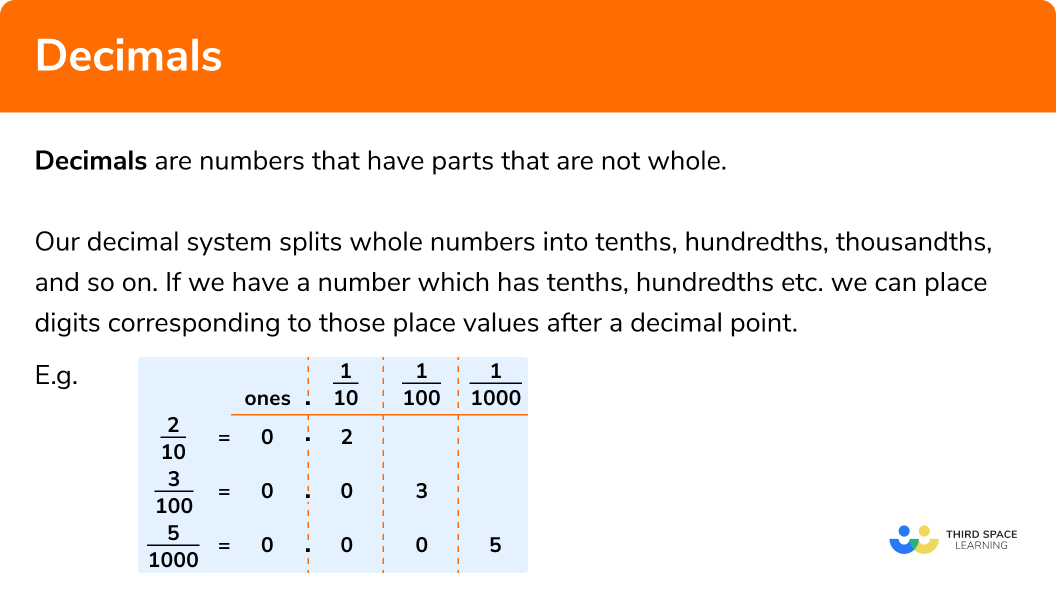## Ordering decimals

You will sometimes be required to compare the size of two decimal numbers or write a list of decimal numbers in order of size.

Ascending order means from smallest to largest while descending order means from largest to smallest.

Let’s look at two techniques that can be used to help order decimals.

• Extra zeros
Another helpful method is to write extra zeros on the end of the decimals so that all numbers have the same amount of decimal places. It can also aid comparison to write the numbers in a vertical line.

For example, which of the following numbers is the largest, 0.08, 0.4, 0.32?

Here we will put a zero on the end of 0.4 so that all the numbers have two decimal places and write them in a vertical line.

0.08

0.40

0.32

Looking down the column of the first decimal place we can see that 4 is the highest digit. Therefore 0.4 is the largest number on this list.

• Compare each place value
It can be helpful to compare one decimal place at a time. This should be done starting with the tenths and grouping the numbers accordingly, before moving on to comparing the next decimal place.

For example, let’s put the following numbers in ascending order: 0.5, 0.2, 0.25, 0.52, 0.05, 0.02, 0.052, 0.025, 0.205, 0.502

All of the numbers have zero units, so we will start by comparing the first decimal place and grouping the numbers accordingly.

Numbers starting 0.0Numbers starting 0.2Numbers starting 0.5
0.05 0.2 0.50
0.020.250 0.520
0.0520.205 0.502
0.025

Now within each group we will look at the subsequent decimal places to order them from smallest to largest.

Numbers starting 0.0Numbers starting 0.2Numbers starting 0.5
0.02 0.2 0.5
0.0250.205 0.502
0.050.25 0.52
0.052

So the final answer is: 0.02, 0.025, 0.05, 0.052, 0.2, 0.205, 0.25, 0.5, 0.502, 0.52.

Step-by-step guide: Decimal place value

## Number line decimal

It can be helpful to visualise decimals on a number line. For example, this number line goes from zero to one in steps of 0.1.

You could be asked to mark a decimal number on a number line.
Here the number 0.472 has been marked. Its position has been estimated between 0.4 and 0.5, but closer to 0.5.

If we zoom in and consider a number line between 0.4 and 0.5 that goes up in steps on 0.01 then we can position the decimal 0.472 more accurately. This time the position has been estimated between 0.47 and 0.48, but closer to 0.47.

If we zoom in once more and consider a number line between 0.47 and 0.48 that goes up in steps on 0.001 then we can mark the decimal 0.472 in the exact position.

All the scales above were marked with ten intervals and therefore went up in steps of 0.1, 0.01 and 0.001. Some decimal scales have a different number of intervals and you may need to calculate the interval steps and mark the scale.

To calculate the length of an interval step we can use the formula:

(\text{Top of the scale} - \text{Bottom of the scale}) \div \text{Number of intervals}

Let’s look at a few examples,

Example 1

This scale goes from 2 to 3 with 5 interval steps. We can use the formula to calculate the length of the interval steps.

(3 - 2) \div 5 = 0.2

So to mark each interval we add 0.2 each time starting with the number at the bottom of the scale; the number 2 in this case.

2+0.2 = 2.2

2.2+0.2=2.4

2.4+0.2=2.6

2.6+0.2=2.8

2.8+0.2= 3

Example 2

First we use the formula to find the length of the interval steps.

(13 - 9) \div 8 = 0.5

Then we can mark each interval by adding 0.5 each time starting with the number at the bottom of the scale.

Example 3

To calculate the interval steps we do (17 - 15) \div 5 = 0.4 Then we add 0.4 to 15 to mark the first interval and continue this process until we reach 17.

Step-by-step guide: Decimal number line

### Calculations with decimals

Each method of decimal arithmetic is summarised below. For detailed examples, practice questions and worksheets on each one follow the links to the step by step guides.

We can apply the four operations to decimal numbers. To do this we can apply our usual adding, subtracting, multiplying and dividing methods with a few additional steps.

• Decimal subtraction

Step-by-step guide: Adding and subtracting decimals

• Decimal multiplication

• Decimal division

Step-by-step guide: Multiplying and dividing decimals

E.g. Work out

$5.68 + 7.8$

1Set up a column addition, writing one number above the other. Make sure the decimal points line up to ensure numbers in each column have the correct place value.

2Add each column from right to left remembering to carry numbers to the next column when you get a two digit number.

### How to add decimals in 3 steps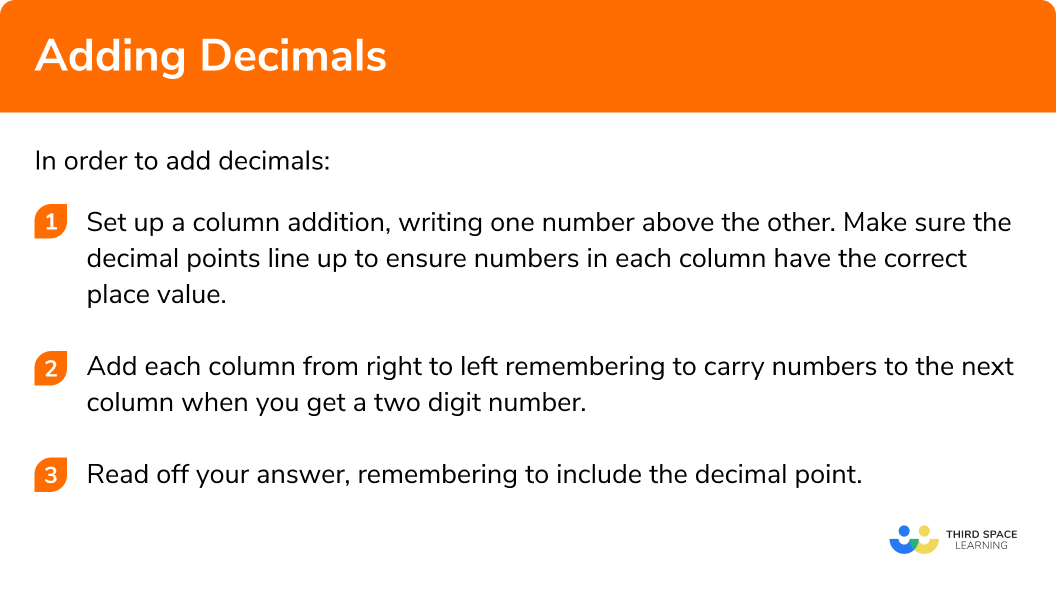## How to subtract decimals

E.g. Work out

$7.5 – 3.81$

1Set up a column subtraction, writing the larger number above the other. Make sure the decimal points line up to ensure numbers in each column have the correct place value. Fill in any gaps with zeros.

2Subtract each column from right to left remembering to exchange (or borrow) from the left if required.

3Read off your answer, remembering to include the decimal point. If the original subtraction would have given a negative answer, remember to include the negative sign.

### Explain how to subtract decimals in steps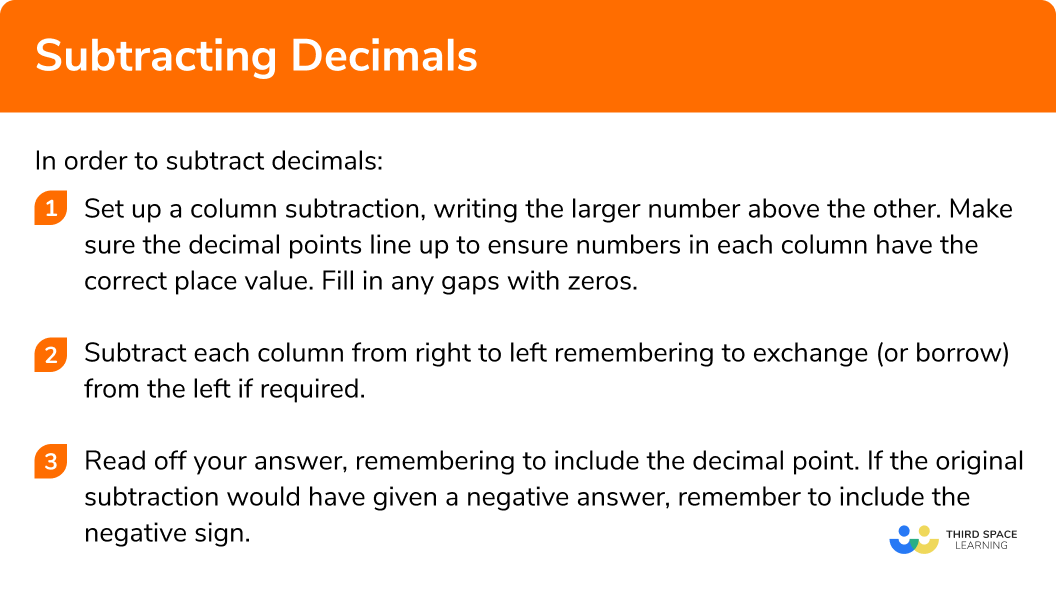## How to multiply decimals

E.g. Work out

$5.3\times4.6$

1Multiply any decimal numbers by an appropriate power of ten to make them whole numbers.

Multiply 5.3 by 10 to make it 53 and multiply 4.6 by 10 to make it 46.

2Use column multiplication (or another preferred method) to find the product of the numbers.

$53\times46 = 2438$

3Divide the product from Step 2 by the powers of ten used in Step 1. Check that the total number of decimal digits in the question is the same as the total number of decimal digits in the answer.

In Step 1 we multiplied 5.3 by 10 and 4.6 by 10.
10 × 10 = 100 so now divide by 100.

$2438\div100 = 24.38$

Check that the total number of decimals in the question matches the total number of decimals in the answer.

### Explain how to multiply decimals in 3 steps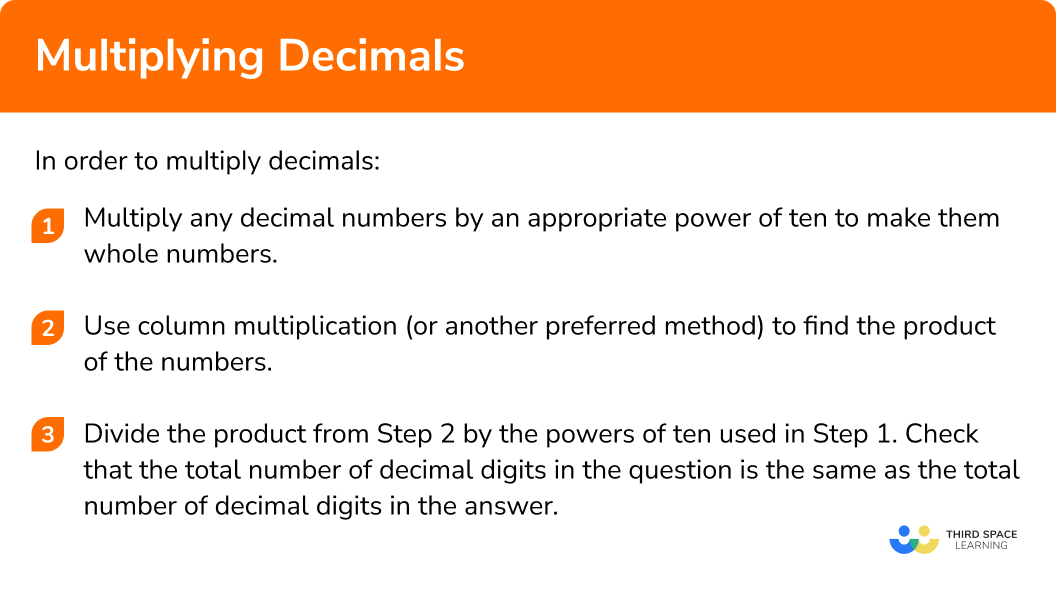## How to divide decimals

E.g. Work out

$27.12\div0.6$

1If the divisor is already an integer, you can go straight to step 3. Otherwise, write the division as a fraction with the divisor as the denominator of the fraction.

2Multiply both the numerator and denominator by the same power of 10 (or other appropriate value) to get an equivalent fraction with an integer denominator. (You can simplify the fraction further if possible to make the division easier to calculate.)

3Use short division to calculate the answer to the original division problem. If the dividend is a decimal, line up the decimal point for your quotient answer and you may need to add some trailing zeros whilst doing the division to get the correct number of decimal places.)

### Explain how to divide decimals in 3 steps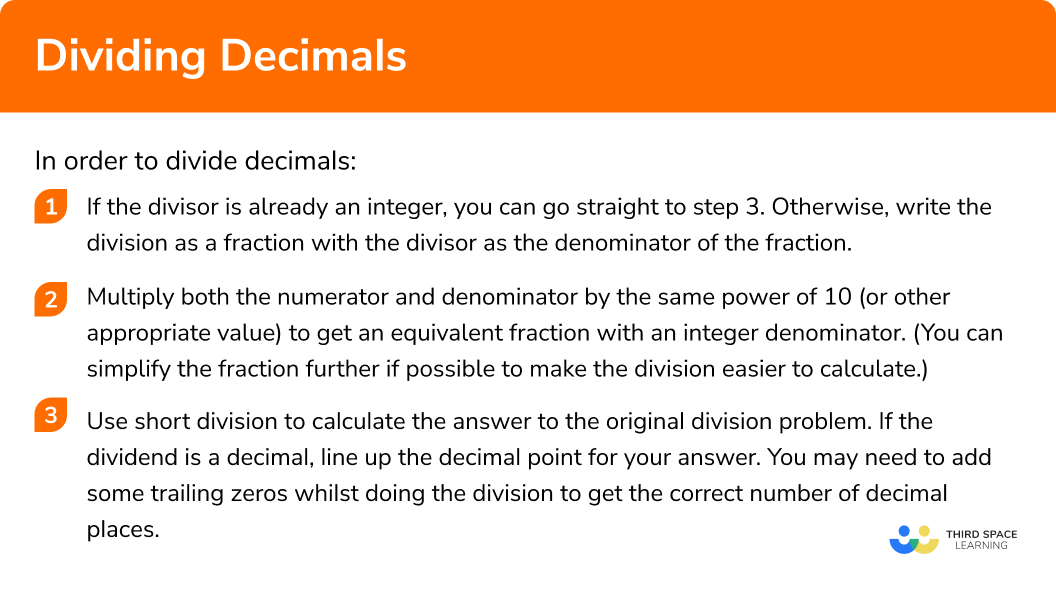### Practice decimals questions

1. Work out 4.79 + 7.83

12.6211.6211.16211.52You must remember to line up the decimal points carefully and carry to the next column if the sum of the digits gives a two digit answer.

2. Work out 14.39 – 9.48

5.95.095.114.91You must remember to line up the decimal points carefully and exchange (or borrow) from the left if required.

3. Multiply together 4.6 and 12.7

584258.4248.4217.3Work out 46\times127 and then divide the answer by 100 .

4. Find the product of 5.13 and 4.9

251.3720.11725.13725137Work out 513\times49 and then divide the answer by 1000 .

5. Work out 12\div0.8

150151.51500Multiply both by 10 , then use short division.

6. Work out 10.2\div0.15

686.86800.68Multiply both by 100 , simplify the fraction by dividing both dividend and divisor by 5, then use short division.

### Decimals GCSE questions

1. You are given that 156 \times 249=38844 .
Use this information to work out the following:

(a) 15.6 \times 2.49

(b) 0.156 \times 24.9

(c) 388.44 \div 2.49

(3 marks)

(a) 38.844

(1)

(b) 3.8844

(1)

(c) 156

(1)

2. (a) If F=ma , find F , when m=4.8 and a=1.75 .

(b) If D=\frac{t}{2}-g , find D , when t=6.5 and g=1.428

(4 marks)

(a) 4.8 \times 1.75

(1)

=8.4

(1)

(b) 6.5 \div 2 (=3.25)

(1)

(1)

3. Below is the breakfast menu for a hotel.The Smith family ordered one cooked buffet, one vegetarian buffet, three continentals, three teas and two coffees. Calculate their total bill.

(3 marks)

Calculating three teas (£ 3.60 ) or two coffees (£ 3.18 ) or three continentals (£ 14.25 )

(1)

Method to add cost of items

(1)

Correct total of £ 37.63

(1)

## Learning checklist

You have now learned how to:

• Understand and use place value for decimals, measures and integers of any size
• Use the four operations, including formal written methods, applied to integers, decimals, proper and improper fractions, and mixed numbers, all both positive and negative

## Still stuck?

Prepare your KS4 students for maths GCSEs success with Third Space Learning. Weekly online one to one GCSE maths revision lessons delivered by expert maths tutors.

Find out more about our GCSE maths tuition programme.